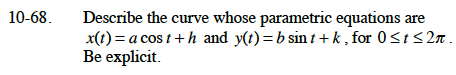### Home > CALC > Chapter 10 > Lesson 10.1.7 > Problem10-68

10-68.

Describe the curve whose parametric equations are x(t) = a cos t + h and y(t) = b sin t + k, for 0 ≤ t ≤ 2π. Be explicit. Homework Help ✎